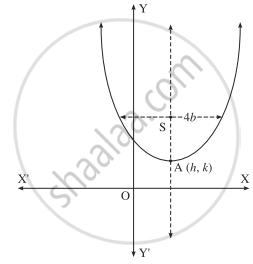# Form the differential equation of all parabolas which have 4b as latus rectum and whose axis is parallel to Y-axis. - Mathematics and Statistics

Sum

Form the differential equation of all parabolas which have 4b as latus rectum and whose axis is parallel to the Y-axis.

#### Solution

Let A(h, k) be the vertex of the parabola which has 4b as a latus rectum and whose axis is parallel to Y-axis. Then the equation of the parabola is

(x - h)2 = 4b(y - k)    .....(1)

where h and k are arbitrary constants.Differentiating both sides of (1) w.r.t. x, we get

2("x - h") * "d"/"dx" ("x - h") = "4b""d"/"dx" ("y - k")

∴ 2("x - h") xx (1 - 0) = "4b"("dy"/"dx" - 0)

∴ (x - h) = 2b"dy"/"dx"

Differentiating again w.r.t. x, we get

1 - 0 = "2b"("d"^2"y")/"dx"^2

∴ "2b"("d"^2"y")/"dx"^2 - 1 = 0

This is the required D.E.

Concept: Formation of Differential Equations
Is there an error in this question or solution?
Chapter 6: Differential Equations - Miscellaneous exercise 2 [Page 217]

#### APPEARS IN

Balbharati Mathematics and Statistics 2 (Arts and Science) 12th Standard HSC Maharashtra State Board
Chapter 6 Differential Equations
Miscellaneous exercise 2 | Q 4.2 | Page 217
Share